```SELECTED PRINCIPLES: ELECTROCHEMICAL CELLS
An electrochemical cell transduces chemical energy to electrical energy
when it is part of a complete electrical circuit.
An exothermic reaction occurs when a piece of zinc metal is placed in
aqueous copper(II) sulfate; i.e.,``````In this redox reaction, electrons are transferred from zinc atoms to
copper(II) ions because zinc is a better reducing agent (i.e., it is
more easily oxidized); the redox half equations are:```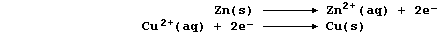```In such an experiment, the energy transduced is released as heat energy
because the electrons are transferred directly from the reductant to
the oxidant in solution. However, when the reductant is physically
separated from the oxidant, then the electrons can be transferred via
an external circuit; and so, as a reaction proceeds, chemical energy
will be transduced to electrical energy.
An electrochemical cell is constructed by physically separating the
reductant from the oxidant; a typical example, connected to an ammeter
and a filament lamp, is shown in the diagram below.```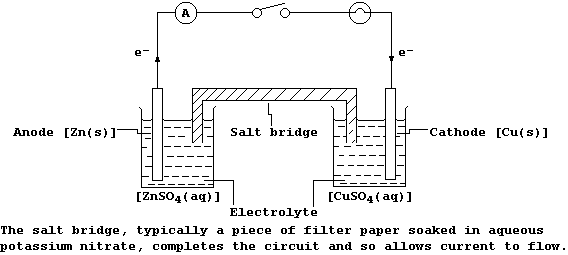```When the switch is closed, the ammeter registers a reading and the lamp
lights; these observations are interpreted as follows.
At the anode, zinc atoms lose two electrons, and so the mass of zinc
decreases and the concentration of aqueous zinc ions increases; i.e.,```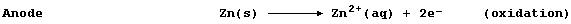```These electrons flow through the wire of the external circuit - where
their electrical energy is transduced to heat and light energy of the
filament lamp - to the copper electrode. At this cathode, copper(II)
ions gain two electrons, and so the concentration of aqueous copper(II)
ions decreases and the mass of copper increases; i.e., ``````Finally, if both the ammeter and the lamp are replaced by a voltmeter,
then the above cell will register its maximum potential difference (or
voltage); this value is +1.10 V, when each solution has a concentration
of 1.00 mol dm-ł and the temperature is 25°C (298 K).``````1.  The energy change (DE) which occurs when a cell transduces chemical
to electrical energy can be calculated using this equation,
DE = - n × F × V
where: n is the number of electrons transferred; F is a constant, with
a value of 96500 C mol-ą; and, V is the potential difference (p.d.).
Complete the Table below, using this equation to calculate the energy
change for each cell; the number of electrons transferred, n, is two.```
 ` Anode ` ` Cathode ` ` Cell p.d. / V ` ` Energy change (DE) / kJ mol-ą` ` Mg ` ` Cu ` ` 2.70 ` ` ` ` Mg ` ` Fe ` ` 1.92 ` ` ` ` Zn ` ` Cu ` ` 1.10 ` ` ` ` Fe ` ` Cu ` ` 0.78` ` ` ` Zn ` ` Fe ` ` 0.32 ` ` ` ` Cu ` ` Cu ` ` 0.00 ` ` `
```                                                                    
2.  A fuel cell is an electrochemical cell in which the reactants are
supplied from outside (rather than forming an integral part of its
construction); a typical example, that of a hydrogen-oxygen fuel cell,
is shown in the diagram below. ```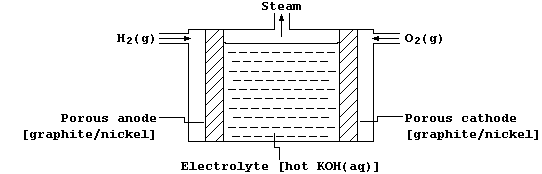```The overall reaction in this fuel cell is identical to the combustion
of dihydrogen; i.e.,```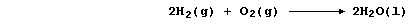```However, the oxidation and reduction are carried out separately at the
anode and cathode, respectively; i.e.,```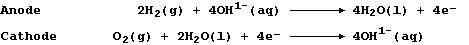```The graphite/nickel electrodes have two purposes: first, they serve as
electrical conductors; and second, they provide the necessary surfaces
for the dissociation of molecules into atoms, with nickel acting as an
electrocatalyst, prior to electron transfer.
(a) The symbol equation for the complete combustion of propane is:``````This redox reaction can be carried out in a fuel cell using platinum as
the electrocatalyst. Noting this symbol equation, and the equation for
the anodic reaction, construct the equation for the cathodic reaction.``````Cathode _______________________________________________________________

(b) Properly designed fuel cells are about 70% efficent and are free of
noise and thermal pollution. Despite these attractive features, fuel
cells are not yet in use for the large-scale generation of electrical
energy because of two unresolved (and related) difficulties: first, the
unavailability of suitable electrocatalysts able to function for long
periods of time without being 'poisoned'; and second, that of obtaining
a supply of sufficiently pure fuels. Suggest and explain how impurities
reduce the efficiency of such catalysts. ______________________________
_______________________________________________________________________
_______________________________________________________________________

Dr. R. Peters                                            Contents' List
Organic Chemistry - Introduction (1)          Metals - Introduction (1)```